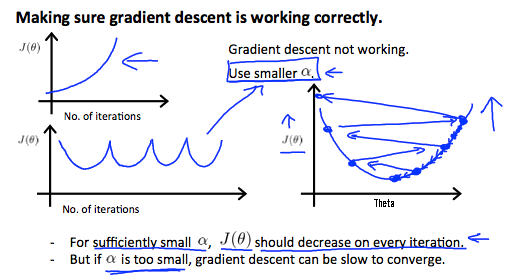# 多变量的线性回归——Linear Regresssion with Multiple Variables

## 多变量线性回归——Multivariant Linear Regression

### 多特征——Multiple Feature

• Notation

$n$$n$ = number of features.

${x}^{\left(i\right)}$$x^{(i)}$ = input of ${i}^{th}$$i^{th}$ training example.

${x}_{j}^{\left(i\right)}$$x_j^{(i)}$ = value of feature j in ${i}^{th}$$i^{th}$ training example.

• Hypothesis

$Previously:{h}_{\theta }\left(x\right)={\theta }_{0}+{\theta }_{1}x$

$Now:{h}_{\theta }\left(x\right)={\theta }_{0}+{\theta }_{1}{x}_{1}+{\theta }_{2}{x}_{2}+...+{\theta }_{n}{x}_{n}$

为了符号的收敛，定义${x}_{0}=1$$x_0 = 1$$\left({x}_{0}^{\left(i\right)}=1\right)$$(x_0^{(i)}=1)$

${h}_{\theta }\left(x\right)=\left[\begin{array}{ccccccc}{\theta }_{0}& {\theta }_{1}& {\theta }_{2}& .& .& .& {\theta }_{n}\end{array}\right]\left[\begin{array}{c}{x}_{0}\\ {x}_{1}\\ {x}_{2}\\ .\\ .\\ .\\ {x}_{n}\end{array}\right]$

$={\theta }^{T}x$

so the hypothsis can be writen:

${h}_{\theta }\left(x\right)={\theta }_{0}{x}_{0}+{\theta }_{1}{x}_{1}+...+{\theta }_{n}{x}_{n}$$h_\theta(x) = \theta_0x_0 + \theta_1x_1 + ... + \theta_nx_n$

$={\theta }^{T}x$$= \theta^T x$

Multivariate Linear Regression

### 多元变量的梯度下降——Gradient Descent for Multiple Variables

repeat until convergence:{

${\theta }_{0}:={\theta }_{0}-\alpha \frac{1}{m}\sum _{i=1}^{m}\left({h}_{\theta }\left({x}^{\left(i\right)}\right)-{y}^{\left(i\right)}\right)\cdot {x}_{0}^{\left(i\right)}$$\theta_0 := \theta_0 - \alpha \frac{1}{m}\sum_{i=1}^m(h_\theta(x^{(i)})-y^{(i)}) \cdot x_0^{(i)}$
${\theta }_{1}:={\theta }_{0}-\alpha \frac{1}{m}\sum _{i=1}^{m}\left({h}_{\theta }\left({x}^{\left(i\right)}\right)-{y}^{\left(i\right)}\right)\cdot {x}_{1}^{\left(i\right)}$$\theta_1 := \theta_0 - \alpha \frac{1}{m}\sum_{i=1}^m(h_\theta(x^{(i)})-y^{(i)}) \cdot x_1^{(i)}$
${\theta }_{2}:={\theta }_{0}-\alpha \frac{1}{m}\sum _{i=1}^{m}\left({h}_{\theta }\left({x}^{\left(i\right)}\right)-{y}^{\left(i\right)}\right)\cdot {x}_{2}^{\left(i\right)}$$\theta_2 := \theta_0 - \alpha \frac{1}{m}\sum_{i=1}^m(h_\theta(x^{(i)})-y^{(i)}) \cdot x_2^{(i)}$

}

repeat until convergence：{

$\theta_j := \theta_0 - \alpha \frac{1}{m}\sum_{i=1}^m(h_\theta(x^{(i)})-y^{(i)}) \cdot x_j^{(i)} \space \space for j := 0...n$
}

### 梯度下降实用技巧（特征缩放)——Gradient Descent in Practice (Feature Scaling)

${x}_{i}:=\frac{{x}_{i}-{\mu }_{i}}{{s}_{i}}$$x_i := \frac{x_i - \mu_i}{s_i}$

${x}_{i}:=\frac{price-1000}{1900}$$x_i := \frac{price-1000}{1900}$

### 特征下降实用技巧（学习率）——Gradient Descent in Practice（Learning rate）

${\theta }_{j}:={\theta }_{j}-\alpha \frac{\mathrm{\partial }}{\mathrm{\partial }{\theta }_{j}}J\left(\theta \right)$$\theta_j := \theta_j - \alpha \frac{\partial}{\partial \theta_j}J(\theta)$

• “Debugging”: How to mark sure gradient descent is working correctly.

• How to choose learning rate $\alpha$$\alpha$

$\alpha$$\alpha$的情况：- 如果 $\alpha$$\alpha$太小：很慢的收敛
- 如果 $\alpha$$\alpha$太大：每个迭代并不减少，并且不收敛